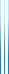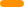# PublicationsPapers

## On the sum of $L_1$ influences of bounded functions

Yuval Filmus, Hamed Hatami, Nathan Keller and Noam Lifshitz
Israel Journal of Mathematics, Volume 214, Issue 1, 2016, pp. 167–192

It is well-known that if $f$ is a Boolean function of degree $d$ then its total influence is bounded by $d$. There are several ways of extending the definition of influence for non-Boolean functions. The usual way is to define the influence of the $i$th variable as the $L_2$ norm of the discrete derivative in direction $i$. Under this definition, the total influence of a bounded function (bounded by 1 in magnitude) is still upper-bounded by the degree.

Aaronson and Ambainis asked whether total $L_1$ influence can be bounded polynomially by the degree, and this was answered affirmatively by Bačkurs and Bavarian, who showed an upper bound of $O(d^3)$ for general functions, and $O(d^2)$ for homogeneous functions. We improve their results by giving an upper bound of $d^2$ in the general case and $O(d\log d)$ in the homogenous case. Our proofs are also much simpler. We also give an almost optimal bound for monotone functions, $d/2\pi + o(d)$.

## BibTeX

@article{FHKL2016,
author = {Yuval Filmus and Hamed Hatami and Nathan Keller and Noam Lifshitz},
title = {Bounds on the sum of {L1} influences},
journal = {Israel Journal of Matematics},
volume = {214},
number = {1},
year = {2016},
pages = {167--192}
}copy to clipboard